NEET  >  31 Year NEET Previous Year Questions: Nuclei - 2

# 31 Year NEET Previous Year Questions: Nuclei - 2

Test Description

## 30 Questions MCQ Test Physics Class 12 | 31 Year NEET Previous Year Questions: Nuclei - 2

31 Year NEET Previous Year Questions: Nuclei - 2 for NEET 2023 is part of Physics Class 12 preparation. The 31 Year NEET Previous Year Questions: Nuclei - 2 questions and answers have been prepared according to the NEET exam syllabus.The 31 Year NEET Previous Year Questions: Nuclei - 2 MCQs are made for NEET 2023 Exam. Find important definitions, questions, notes, meanings, examples, exercises, MCQs and online tests for 31 Year NEET Previous Year Questions: Nuclei - 2 below.
Solutions of 31 Year NEET Previous Year Questions: Nuclei - 2 questions in English are available as part of our Physics Class 12 for NEET & 31 Year NEET Previous Year Questions: Nuclei - 2 solutions in Hindi for Physics Class 12 course. Download more important topics, notes, lectures and mock test series for NEET Exam by signing up for free. Attempt 31 Year NEET Previous Year Questions: Nuclei - 2 | 30 questions in 60 minutes | Mock test for NEET preparation | Free important questions MCQ to study Physics Class 12 for NEET Exam | Download free PDF with solutions
 1 Crore+ students have signed up on EduRev. Have you?
31 Year NEET Previous Year Questions: Nuclei - 2 - Question 1

### The activity of a radioactive sample is measuredas 9750 counts per minute at t = 0 and as 975counts per minute at t = 5 minutes. The decayconstant is approximately 

Detailed Solution for 31 Year NEET Previous Year Questions: Nuclei - 2 - Question 1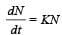9750 = KN0 ............. (1)
975 = KN ............. (2)
Dividing (1) by (2)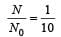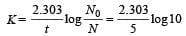= 0.4606 = 0.461 per minute

31 Year NEET Previous Year Questions: Nuclei - 2 - Question 2

### The stable nucleus that has a radius half that of Fe56 is 

Detailed Solution for 31 Year NEET Previous Year Questions: Nuclei - 2 - Question 2

The nuclear radius R = RoA1/3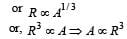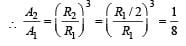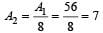31 Year NEET Previous Year Questions: Nuclei - 2 - Question 3

### In a fission reaction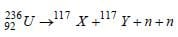the binding energy per nucleon of X and Y is 8.5MeV whereas of 236U is 7.6 MeV. The totalenergy liberated will be about 

Detailed Solution for 31 Year NEET Previous Year Questions: Nuclei - 2 - Question 3

Binding energy
= 117 × 8.5 + 117 × 8.5 – 236 × 7.6
= 234 × 8.5 – 236 × 7.6
= 1989 – 1793.6 = 200 MeV
Thus, in per fission of Uranium nearly 200
MeV energy is liberated

31 Year NEET Previous Year Questions: Nuclei - 2 - Question 4

A free neutron decays into a proton, an electronand 

Detailed Solution for 31 Year NEET Previous Year Questions: Nuclei - 2 - Question 4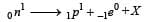X must have zero charge and almost zero mass as electron  is emitted. Hence X must be antineutrino.

31 Year NEET Previous Year Questions: Nuclei - 2 - Question 5

The most penetrating radiation of the following is       

Detailed Solution for 31 Year NEET Previous Year Questions: Nuclei - 2 - Question 5

The penetrating power of radiation is directly proportional to the energy of its photon.
Energy of a photon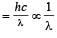∴ Penetrating power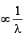λ is minimum for γ-rays, so penetrating power is maximum of γ-rays.

31 Year NEET Previous Year Questions: Nuclei - 2 - Question 6

Which of the following is used as a moderator innuclear reactors? 

Detailed Solution for 31 Year NEET Previous Year Questions: Nuclei - 2 - Question 6

Moderator used in nuclear r eactor are graphite and heavy water.

31 Year NEET Previous Year Questions: Nuclei - 2 - Question 7

Half-lives of two radioactive substances A and B are respectively 20 minutes and 40 minutes.Initially, the samples of A and B have equalnumber of nuclei. After 80 minutes the ratio ofremaining numbers of A and B nuclei is 

Detailed Solution for 31 Year NEET Previous Year Questions: Nuclei - 2 - Question 7

80 = 20 × nA ⇒ nA = 4
80 = 40 × nB ⇒ nB = 2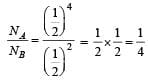31 Year NEET Previous Year Questions: Nuclei - 2 - Question 8

Atomic weight of Boron is 10.81 and it has two isotopes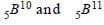. Then the ratio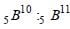in nature would be        

Detailed Solution for 31 Year NEET Previous Year Questions: Nuclei - 2 - Question 8

Suppose that, The number of 10B type atoms = x and the number of 11B type atoms = y
Weight of 10B type atoms = 10x
Weight of 11B type atoms = 11y
Total number of atoms = x + y

∴  Atomic weight⇒10x + 11y = 10.81x + 10.81y
⇒ 0.81x = 0.19y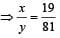31 Year NEET Previous Year Questions: Nuclei - 2 - Question 9

A nucleus nXm emits one α and two β particles. The resulting nucleus is

Detailed Solution for 31 Year NEET Previous Year Questions: Nuclei - 2 - Question 9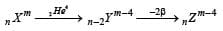31 Year NEET Previous Year Questions: Nuclei - 2 - Question 10

Complete the equation for the following fission process :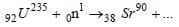Detailed Solution for 31 Year NEET Previous Year Questions: Nuclei - 2 - Question 10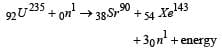31 Year NEET Previous Year Questions: Nuclei - 2 - Question 11

Alpha-particles are 

Detailed Solution for 31 Year NEET Previous Year Questions: Nuclei - 2 - Question 11

We know that alpha particles are the nucleus of ionized helium atoms which contain two protons and two neutrons. These are emitted by the nuclei of certain radioactive substances. Streams of alpha particles, called α-rays, produce intense ionisation in gases through which they pass and are easily absorbed by matter.

31 Year NEET Previous Year Questions: Nuclei - 2 - Question 12

After 1α and 2 β-emissions 

Detailed Solution for 31 Year NEET Previous Year Questions: Nuclei - 2 - Question 12

Emission of 1α particle led to decrease in atomic number by 2 while mass number by 4.
On the other hand, emission of 2β particles increases  atomic number by 2. Hence, overall emission of, 1α and 2β particles led to decrease in mass number by 4.

31 Year NEET Previous Year Questions: Nuclei - 2 - Question 13

The decay constant (λ) and the half-life (T) of a radioactive isotope are related as 

Detailed Solution for 31 Year NEET Previous Year Questions: Nuclei - 2 - Question 13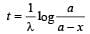when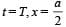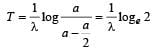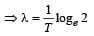31 Year NEET Previous Year Questions: Nuclei - 2 - Question 14

Atomic hydrogen has life period of 

Detailed Solution for 31 Year NEET Previous Year Questions: Nuclei - 2 - Question 14

Atomic hydrogen is unstable and it has life period of a fraction of a second.

31 Year NEET Previous Year Questions: Nuclei - 2 - Question 15

It is possible to understand nuclear fission onthe basis of the 

Detailed Solution for 31 Year NEET Previous Year Questions: Nuclei - 2 - Question 15

According to liquid drop model of nucleus, an excited nucleus breaks into lighter nuclei just like an excited drop breaks into tiny drops.

31 Year NEET Previous Year Questions: Nuclei - 2 - Question 16

In the following nuclear reaction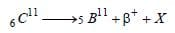what does X stand for? 

Detailed Solution for 31 Year NEET Previous Year Questions: Nuclei - 2 - Question 16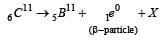X is a neutrino, when β-particle is emitted.

31 Year NEET Previous Year Questions: Nuclei - 2 - Question 17

Mn and Mp represent mass of neutron and protonrespectively. If an element having atomic massM has N-neutron and Z-proton, then the correctrelation will be 

Detailed Solution for 31 Year NEET Previous Year Questions: Nuclei - 2 - Question 17

Given : Mass of neutron = Mn Mass of proton = Mp; Atomic mass of the element = M ;
Number of neutrons in the element = N and number of protons in the element = Z.
We know that the atomic mass (M) of any stable nucleus is always less than the sum of the masses of the constituent particles.
Therefore, M < [NMn + ZMp].

31 Year NEET Previous Year Questions: Nuclei - 2 - Question 18

A sample has 4 × 1016 radioactive nuclei of halflife 10 days. The number of atoms decaying in 30 days is 

Detailed Solution for 31 Year NEET Previous Year Questions: Nuclei - 2 - Question 18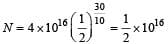Atoms decayed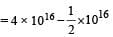= 3.5 × 1016

31 Year NEET Previous Year Questions: Nuclei - 2 - Question 19

A deuteron strikes 8O16 nucleus with subsequent emission of an alpha particle. Identify the nucleus so produced 

Detailed Solution for 31 Year NEET Previous Year Questions: Nuclei - 2 - Question 19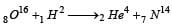31 Year NEET Previous Year Questions: Nuclei - 2 - Question 20

For a nuclear fusion process, the suitable nucleiare 

Detailed Solution for 31 Year NEET Previous Year Questions: Nuclei - 2 - Question 20

For nuclear fusion process the nuclei with low mass are suitable.

31 Year NEET Previous Year Questions: Nuclei - 2 - Question 21

Solar energy is mainly caused due to 

Detailed Solution for 31 Year NEET Previous Year Questions: Nuclei - 2 - Question 21

As a result of fusion, enormous amount of heat is liberated which is the main cause of source of solar energy.

31 Year NEET Previous Year Questions: Nuclei - 2 - Question 22

A nuclear reaction is given by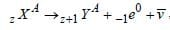represents

Detailed Solution for 31 Year NEET Previous Year Questions: Nuclei - 2 - Question 22

-1e0 represents a β-decay..

31 Year NEET Previous Year Questions: Nuclei - 2 - Question 23

A sample of radioactive element has a mass of 10gm at an instant t=0. The approximate mass ofthis element in the sample after two mean lives is 

Detailed Solution for 31 Year NEET Previous Year Questions: Nuclei - 2 - Question 23

Using the relation for mean life.
Given :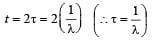Then from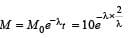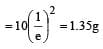31 Year NEET Previous Year Questions: Nuclei - 2 - Question 24

The mass number of a nucleus is 

Detailed Solution for 31 Year NEET Previous Year Questions: Nuclei - 2 - Question 24

In case of hydrogen atom, Mass number = atomic number

31 Year NEET Previous Year Questions: Nuclei - 2 - Question 25

The mass of proton is 1.0073 u and that ofneutron is 1.0087 u (u = atomic mass unit). Thebinding energy of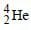is    

Detailed Solution for 31 Year NEET Previous Year Questions: Nuclei - 2 - Question 25

Δm = (2 × 1.0074 + 2 × 1.0087 – 4.0015) = 0.0307
E = (Δm) × 931 MeV = 0.0307 × 931 = 28.5 MeV

31 Year NEET Previous Year Questions: Nuclei - 2 - Question 26

The volume occupied by an atom is greater thanthe volume of the nucleus by a factor of about

Detailed Solution for 31 Year NEET Previous Year Questions: Nuclei - 2 - Question 26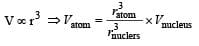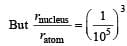Hence, Vatom = 1015 × Vnucleus

31 Year NEET Previous Year Questions: Nuclei - 2 - Question 27

If in nuclear fusion process the masses of thefusing nuclei be m1 and m2 and the mass of theresultant nucleus be m3, then 

Detailed Solution for 31 Year NEET Previous Year Questions: Nuclei - 2 - Question 27

m3 < (m1 + m2) (∵ m1 + m2 = m3 + E ]
as E = [m1 + m2 – m3] C2

31 Year NEET Previous Year Questions: Nuclei - 2 - Question 28

A nucleus represented by the symbol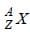has

Detailed Solution for 31 Year NEET Previous Year Questions: Nuclei - 2 - Question 28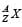has Z protons and (A – Z) neutrons

31 Year NEET Previous Year Questions: Nuclei - 2 - Question 29

Mp denotes the mass of a proton and Mn that ofa neutron. A given nucleus, of binding energyB, contains Z protons and N neutrons. The mass M (N, Z) of the nucleus is given by (c is thevelocity of light) 

Detailed Solution for 31 Year NEET Previous Year Questions: Nuclei - 2 - Question 29

Mass defect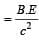Mass of nucleus = Mass of proton + mass of neutron  – mass defect

31 Year NEET Previous Year Questions: Nuclei - 2 - Question 30

The nuclei of which one of the following pairs of nuclei are isotones? 

Detailed Solution for 31 Year NEET Previous Year Questions: Nuclei - 2 - Question 30

Isotones means equal number of neutrons i.e.,   (A–Z) = 74 –34 = 71 – 31 = 40.

## Physics Class 12

157 videos|452 docs|213 tests
 Use Code STAYHOME200 and get INR 200 additional OFF Use Coupon Code
Information about 31 Year NEET Previous Year Questions: Nuclei - 2 Page
In this test you can find the Exam questions for 31 Year NEET Previous Year Questions: Nuclei - 2 solved & explained in the simplest way possible. Besides giving Questions and answers for 31 Year NEET Previous Year Questions: Nuclei - 2, EduRev gives you an ample number of Online tests for practice

## Physics Class 12

157 videos|452 docs|213 tests

### How to Prepare for NEET

Read our guide to prepare for NEET which is created by Toppers & the best Teachers